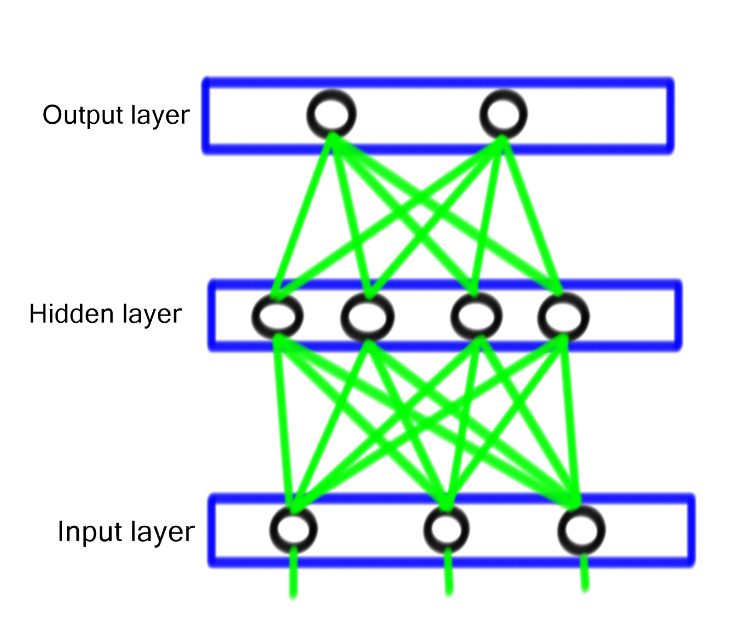## Can my computer read my handwriting? Neural Networks and Genetic Algorithms Part 2

### Putting Neurons together

Now that we have our neurons and understand what they do (not much, actually.  Just some simple multiplication and addition) we can start to organize them into a way that will let the computer read our handwriting.  How do we do that, you ask?  By making them into a neural network.

Neural networks are actually simple concepts also.  They are made up of layers of neurons called neural layers.  Every neural network is going to have at least three layers.  An input layer, an output layer, and one or more hidden layers.  Each of these layers is going to be made up of a number of neurons.This is a simple diagram with three neurons in the input layer, four in the single hidden layer, and two in the output layer.  The green lines represent inputs and outputs and how the data travels between layers.  As you can see, the data passes into each neuron on the layer from the layer before it.  Then the output of the neuron is sent along to the next layer.

### How many neurons do I need?

Determining the number of neurons needed in the input and output layers is straightforward.  In my example, I wanted to be able to recognize the numbers 0 through 9, so I have a total of 10 possible outputs.  So the number of neurons in my output layer is 10.  I will take the output from those 10 neurons and find the highest one.  If the first neuron has the highest output, then I'll assume that the neural network thinks this input is a zero.  The second neuron will be a 1.  The third 2 and so on.  Not coincidentally the output solutions line up with the neurons array position n = 0, n = 1 to make some of the coding easier.

Inputs is a little trickier.  I decided that to start I would with something simple and just read in data from an 8 x 8 grid.  I will have 64 total pixels that can be either on or off, so I need 64 neurons in my input layer.

The hidden layer is tricky.  As I understand it, there isn't a good consensus on how many neurons should be in the hidden layers and, as of this writing, no formula to determine it.  A good rule of thumb is to have the hidden layer have neurons equal to the average of the input and output layers, so I arbitrarily chose 40 neurons for my hidden layer.  At some point I'll change around that number to see what difference it makes, but to be perfecly honest I really don't care.

### What do you mean you don't care?

I don't care how many hidden neurons my neural network has.  All that I care about is that it works.  Which brings me to the hardest part of programing and working with them.  I don't know how they work.  I mean, I conceptually know how they do.  They are simple multiplication and adding machines.  But once I string them together and train them up (we will talk about training in part 3) I don't know how they are getting the correct answer.

This is radically different from traditional programming.  Normally, I take the input and do something with it to generate an output.  Sometimes the output is unexpected, but that is because I as the programmer made a mistake in coding the rules.  With a neural network I am turning over the development of the rules to the network itself.  It is making its own rules and I am just telling it if it is correct or not.

So my computer is making up the rules necessary to get the right anwers and I don't know how it does it.  Creepy!